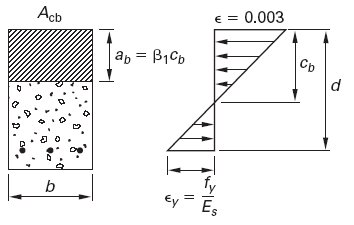Related Resources: calculators

### Maximum Reinforcement Steel Area per. ACI 318 Calculator

Maximum Reinforcement Steel Area per. ACI 318 Equations and Calculator

In a ductile failure mode, the steel yields in tension before the concrete crushes in compression. (The opposite would be a brittle failure mode.) The area of tension steel for which steel yielding occurs simultaneously with concrete crushing in compression is known as the balanced steel area, Asb. An under-reinforced beam is defined as one having a steel area less than Asb. An over-reinforced beam has more steel than Asb.

The balanced steel area is

Eq. 1
Asb = ρsb · b · d = 0.85 · f 'c · Acb / fy = 0.85 · f 'c · ab · b / fy

Asb = 0.85 · f 'c · β1 cb b / fy

Figure 1 illustrates that the compression area for balanced conditions, Acb, extends from the most compressed fiber to a depth equal to ab.

Eq. 2(a), SI
ab = β1 ( 600 / ( 600 + fy ) ) d

Eq. 2(b), US
ab = β1 ( 87,000 / ( 87,000 + fy ) ) d

Figure 1, Beam at Balanced ConditionThe factor β1 is defined as

Eq. 3(a), SI
β1 = 0.85 [ f 'c ≤ 27.6 MPa]

Eq. 3(b), US
β1 = 0.85 [ f 'c ≤ 4,000 lbf/in2]

Eq. 3(c), SI
β1 = 0.85 - 0.05 (( f 'c - 27.6 ) / 6.9 ) ≥ 0.65 MPa

Eq. 3(d), US
β1 = 0.85 - 0.05 (( f 'c - 4,000 ) / 1,000) ≥ 0.65 lbf/in2

Combining Eq. 1 and Eq. 2 with Acb = abb, for rectangular sections, the balanced steel area is

Eq. 4(a), SI
Asb = ρsb · b · d = ( ( 0.85 ·β1 · f 'c ) / fy · (600 / ( 600 + fy ) ) · b · d

Eq. 4(b), US
Asb = ρsb · b · d = ( ( 0.85 · β1 · f 'c ) / fy · (87,000 / ( 87,000 + fy ) ) · b · d

Previous versions of ACI 318 ensured the steel would fail in tension before the concrete failed in compression (i.e., ductile failure) by limiting the maximum reinforcement to 75% of the balanced reinforcement. (This provision is still present in ACI 318 Sec. B10.3.3 of the alternative provisions.)

Eq. 5
As,max = 0.75 · Asb [alternative provisions only]

For unified design, the ductile failure is ensured by specifying a minimum tensile steel strain, εt,min of 0.004.

The unified design provisions limit the maximum reinforcement in a flexural member (with factored axial load less than 0.1f'cAg) to that which would result in a net tensile strain, εt , not less than 0.004 [ACI 318 Sec. 10.3.5]. The fraction of the balanced reinforcement ratio equivalent to a steel strain of 0.004 depends on both the concrete ultimate compressive strength and the steel tensile yield strength. For a singly reinforced section with grade 60 reinforcement, this is equivalent to a maximum reinforcement ratio of 0.714ρb. (By comparison, the ACI 318 App. B limit of ρmax = 0.75ρb results in a net tensile strain of 0.00376.) At the net tensile strain limit of 0.004, the strength reduction factor of ACI 318 Sec. 9.3.2, Φ, is reduced to 0.817.

It is almost always advantageous to limit the net tensile strain in flexural members to a minimum of 0.005, which is equivalent to a maximum reinforcement ratio of 0.63ρb [ACI 318 Sec. R9.3.2.2], even though the code permits higher amounts of reinforcement that produce lower net tensile strains. Where member size is limited and extra strength is needed, it is best to use compression reinforcement to limit the net tensile strain so that the section is tension controlled.

Where:

Asb = Area, in2 (mm2)
ab = depth from equation 2, in (mm)
b = width, in (mm)
cb = distance, in (mm)
d = Beam effective depth (tension to steel centroid), in, (m)
β1 = stress constant, equation 3, lbf/in2 (MPa)
fy = Tension steel yield strength, lbf/in2 (MPa)
f 'c = Compressive strength of concrete, lbf/in2 (MPa)
bw = Width of web, ft, (m)
ρsb = reinforment steel ratio

Reference

• ACI 318 Building Code Requirements for Structural Steel, paragraph 10.5 Minimum reinforcement of flexural members
• Civil Engineering Reference Manual, Fifteenth Edition, Chapter 48, p. 50.6, Paragraph 8 (Maximum Steel Area), Michael R. Lindeburg, PE

Related